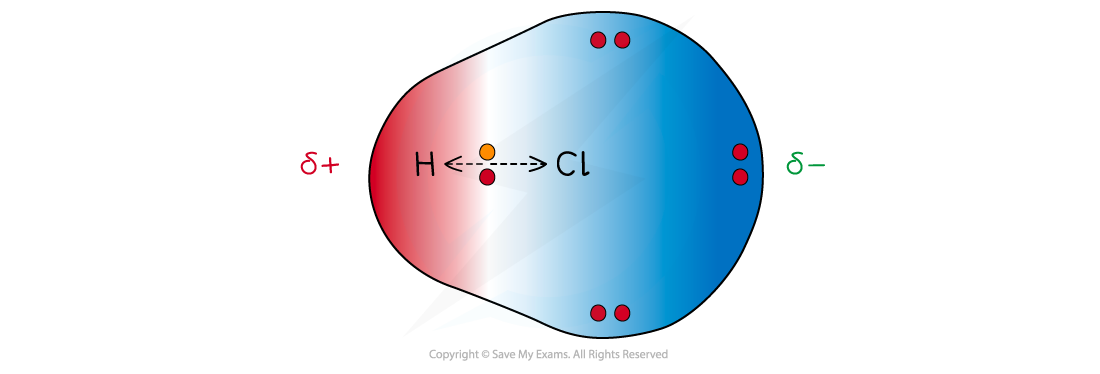# IB DP Chemistry: SL复习笔记4.1.5 Bond Polarity

### Bond Polarity

• When two atoms in a covalent bond have the same electronegativity the covalent bond is nonpolarThe two chlorine atoms have identical electronegativities so the bonding electrons are shared equally between the two atoms

• When two atoms in a covalent bond have different electronegativities the covalent bond is polar and the electrons will be drawn towards the more electronegative atom
• As a result of this:
• The negative charge centre and positive charge centre do not coincide with each other
• This means that the electron distribution is asymmetric
• The less electronegative atom gets a partial charge of δ+ (delta positive)
• The more electronegative atom gets a partial charge of δ- (delta negative)
• The greater the difference in electronegativity the more polar the bond becomesCl has a greater electronegativity than H causing the electrons to be more attracted towards the Cl atom which becomes delta negative and the H delta positive

#### Dipole moment

• The dipole moment is a measure of how polar a bond is
• The direction of the dipole moment is shown by the following sign in which the arrow points to the partially negatively charged end of the dipole:The sign shows the direction of the dipole moment and the arrow points to the delta negative end of the dipole

#### Worked Example

The electronegativity values of four elements are given.

C = 2.6        N = 3.0       O = 3.4       F = 4.0

What is the order of increasing polarity of the bonds in the following compounds?A. CO < OF2 < NO < CF4

B. NO < OF2 < CO < CF4

C. CF4 < CO < OF2 < NO

D. CF4 < NO < OF2 < CO

The correct option is B.

• You have to calculate the difference in electronegativity for the bonds and then rank them from smallest to largest:

NO  (3.4 - 3.0 = 0.4)

OF(4.0 - 3.4 = 0.6)

CO   (3.4 - 2.6 = 0.8)

CF4  (4.0 - 2.6 = 1.4)